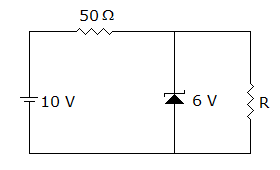# Electronics and Communication Engineering - Electronic Devices and Circuits

36.

In a p-n-p transistor the main current carriers and the mechanism of flow respectively are

 A. electrons, drift B. holes, drift C. holes, diffusion D. electrons, diffusion

Answer: Option C

Explanation:

No answer description available for this question. Let us discuss.

37.

The 6 V zener diode shown in figure has zero zener resistance and a knee current of 5 mA. The minimum value of R, so that the voltage across it does not fall below 6 V isA. 1.2 kΩ B. 80 Ω C. 50 W D. 0

Answer: Option B

Explanation:

No answer description available for this question. Let us discuss.

38.

Secondary emission occurs in

 A. diode B. triode C. tetrode D. pentode

Answer: Option C

Explanation:

No answer description available for this question. Let us discuss.

39.

In common base connection, the output characteristics of a bipolar junction transistor is drawn between

 A. IC and VCB B. IC and VCE C. IE and VCB D. IE and VCE

Answer: Option A

Explanation:

No answer description available for this question. Let us discuss.

40.

In fabricating silicon BJT in ICs by the epitaxial process, the number of diffusions used is usually

 A. 2 B. 3 C. 4 D. 6

Answer: Option D

Explanation:

No answer description available for this question. Let us discuss.

#### Current Affairs 2021

Interview Questions and Answers